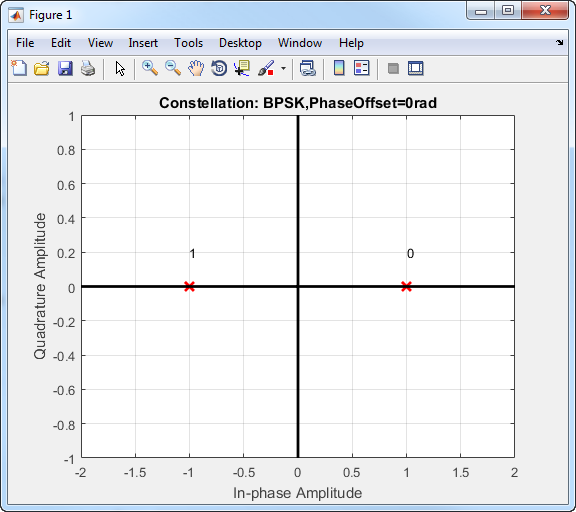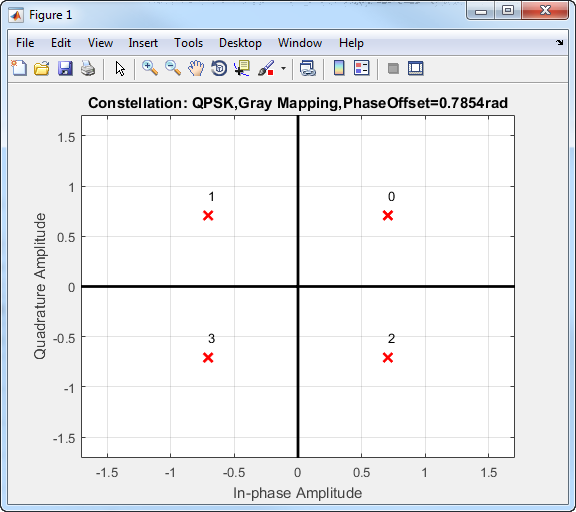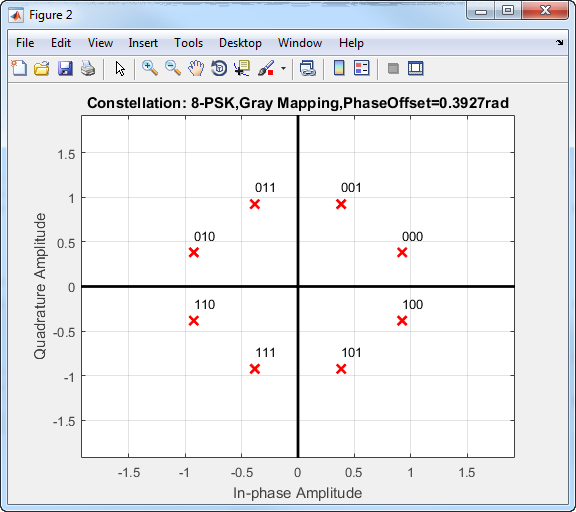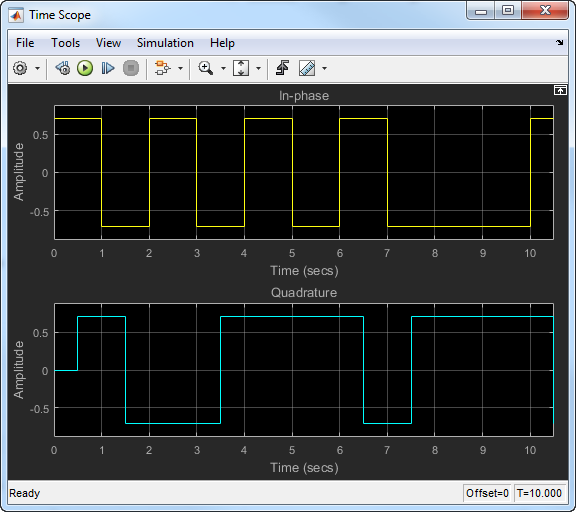Documentation

## Phase Modulation

Phase modulation is a linear baseband modulation technique in which the message modulates the phase of a constant amplitude signal. Communications Toolbox™ provides modulators and demodulators for these phase modulation techniques:

• Phase shift keying (PSK) — Binary, quadrature, and general PSK

• Differential phase shift keying (DPSK) — Binary, quadrature, and general DPSK

• Offset QPSK (OQPSK)To modulate input data with these techniques, you can use MATLAB® functions, System objects, or Simulink® blocks.

Modulation SchemeMATLAB functionsSystem objectsSimulink blocks
Binary PSK (BPSK)
General PSK
Differential BPSK (DBPSK)
Differential QPSK (DQPSK)
General DPSK
OQPSK

### Baseband and Passband Simulation

Communications Toolbox supports baseband and passband simulation methods; however, the phase shift keying techniques support baseband simulation only.

A general passband waveform can be represented as

`${Y}_{1}\left(t\right)\mathrm{cos}\left(2\pi {f}_{c}t+\theta \right)-{Y}_{2}\left(t\right)\mathrm{sin}\left(2\pi {f}_{c}t+\theta \right)\text{\hspace{0.17em}},$`

where fc is the carrier frequency and θ is the initial phase of the carrier signal. This equation is equal to the real part of

`$\left[\left({Y}_{1}\left(t\right)+j{Y}_{2}\left(t\right)\right){e}^{j\theta }\right]\mathrm{exp}\left(j2\pi {f}_{c}t\right)\text{\hspace{0.17em}}.$`

In a baseband simulation, only the expression within the square brackets is modeled. The vector y is a sampling of the complex signal

`$\left({Y}_{1}\left(t\right)+j{Y}_{2}\left(t\right)\right){e}^{j\theta }\text{\hspace{0.17em}}.$`

### BPSK

In binary phase shift keying (BPSK), the phase of a constant amplitude signal switches between two values corresponding to binary 1 and binary 0. The passband waveform of a BPSK signal is

`${s}_{n}\left(t\right)=\sqrt{\frac{2{E}_{b}}{{T}_{b}}}\mathrm{cos}\left(2\pi {f}_{c}t+\pi \left(1-n\right)\right);\text{\hspace{0.17em}}n\in \left\{0,1\right\},$`

where:

• Eb is the energy per bit.

• Tb is the bit duration.

• fc is the carrier frequency.

In MATLAB, the baseband representation of a BPSK signal is

`${s}_{n}\left(t\right)=\mathrm{cos}\left(\pi n\right);\text{\hspace{0.17em}}n\in \left\{0,1\right\}.$`

The BPSK signal has two phases: 0 and π.The probability of a bit error in an AWGN channel is

`${P}_{b}=Q\left(\sqrt{\frac{2{E}_{b}}{{N}_{0}}}\right),$`

where N0 is the noise power spectral density.

### QPSK

In quadrature phase shift keying, the message bits are grouped into 2-bit symbols, which are transmitted as one of four phases of a constant amplitude baseband signal. This grouping provides a bandwidth efficiency that is twice as great as the efficiency of BPSK. The general QPSK signal is expressed as

`${s}_{n}\left(t\right)=\sqrt{\frac{2{E}_{s}}{{T}_{s}}}\mathrm{cos}\left(2\pi {f}_{c}t+\left(2n+1\right)\frac{\pi }{4}\right);\text{ }n\in \left\{0,1,2,3\right\},$`

where Es is the energy per symbol and Ts is the symbol duration. The complex baseband representation of a QPSK signal is

`${s}_{n}\left(t\right)=\mathrm{exp}\left(j\pi \left(\frac{2n+1}{4}\right)\right);\text{ }n\in \left\{0,1,2,3\right\}.$`

In this QPSK constellation diagram, each 2-bit sequence is mapped to one of four possible states. The states correspond to phases of π/4, 3π/4, 5π/4, and 7π/4.To improve bit error rate performance, the incoming bits can be mapped to a Gray-coded ordering.

Binary-to-Gray Mapping

Binary SequenceGray-coded Sequence
0000
0101
1011
1110

The primary advantage of the Gray code is that only one of the two bits changes when moving between adjacent constellation points. Gray codes can be applied to higher-order modulations, as shown in this Gray-coded QPSK constellation.The bit error probability for QPSK in AWGN with Gray coding is

`${P}_{b}=Q\left(\sqrt{\frac{2{E}_{b}}{{N}_{0}}}\right),$`

which is the same as the expression for BPSK. As a result, QPSK provides the same performance with twice the bandwidth efficiency.

### Higher-Order PSK

In MATLAB, you can modulate and demodulate higher-order PSK constellations. The complex baseband form for an M-ary PSK signal using natural binary-ordered symbol mapping is

`${s}_{n}\left(t\right)=\mathrm{exp}\left(j\pi \left(\frac{2n+1}{M}\right)\right);\text{ }n\in \left\{0,1,\dots ,M-1\right\}.$`

This 8-PSK constellation uses Gray-coded symbol mapping.For modulation orders beyond 4, the bit error rate performance of PSK in AWGN worsens. In the following figure, the QPSK and BPSK curves overlap one another.### DPSK

DPSK is a noncoherent form of phase shift keying that does not require a coherent reference signal at the receiver. With DPSK, the difference between successive input symbols is mapped to a specific phase. As an example, for binary DPSK (DBPSK), the modulation scheme operates such that the difference between successive bits is mapped to a binary 0 or 1. When the input bit is 1, the differentially encoded symbol remains the same as the previous symbol, while an incoming 0 toggles the output symbol.

The disadvantage of DPSK is that it is approximately 3 dB less energy efficient than coherent PSK. The bit error probability for DBPSK in AWGN is Pb = 1/2 exp(Eb/N0).

### OQPSK

Offset QPSK is similar to QPSK except that the time alignment of the in-phase and quadrature bit streams differs. In QPSK, the in-phase and quadrature bit streams transition at the same time. In OQPSK, the transitions have an offset of a half-symbol period as shown.The in-phase and quadrature signals transition only on boundaries between symbols. These transitions occur at 1-second intervals because the sample rate is 1 Hz. The following figure shows the in-phase and quadrature signals for an OQPSK signal.For OQPSK, the quadrature signal has a 1/2 symbol period offset (0.5 s).

The BER for an OQPSK signal in AWGN is identical to that of a QPSK signal. The BER is

`${P}_{b}=Q\left(\sqrt{\frac{2{E}_{b}}{{N}_{0}}}\right),$`

where Eb is the energy per bit and N0 is the noise power spectral density.

### Soft-Decision Demodulation

All Communications Toolbox demodulator functions, System objects and blocks can demodulate binary data using either hard decisions or soft decisions. Two soft-decision algorithms are available: exact log-likelihood ratio (LLR) and approximate LLR. Exact LLR provides the greatest accuracy but is slower, while approximate LLR is less accurate but more efficient.

#### Exact LLR Algorithm

The log-likelihood ratio (LLR) is the logarithm of the ratio of probabilities of a 0 bit being transmitted versus a 1 bit being transmitted for a received signal. The LLR for a bit, b, is defined as:

`$L\left(b\right)=\mathrm{log}\left(\frac{\mathrm{Pr}\left(b=0|r=\left(x,y\right)\right)}{\mathrm{Pr}\left(b=1|r=\left(x,y\right)\right)}\right)$`

Assuming equal probability for all symbols, the LLR for an AWGN channel can be expressed as:

`$L\left(b\right)=\mathrm{log}\left(\frac{\sum _{s\in {S}_{0}}{e}^{-\frac{1}{{\sigma }^{2}}\left({\left(x-{s}_{x}\right)}^{2}+{\left(y-{s}_{y}\right)}^{2}\right)}}{\sum _{s\in {S}_{1}}{e}^{-\frac{1}{{\sigma }^{2}}\left({\left(x-{s}_{x}\right)}^{2}+{\left(y-{s}_{y}\right)}^{2}\right)}}\right)$`

VariableDescription

`$r$`

Received signal with coordinates (x, y)

`$b$`

Transmitted bit (one of the K bits in an M-ary symbol, assuming all M symbols are equally probable)

`${S}_{0}$`

Ideal symbols or constellation points with bit 0, at the given bit position

`${S}_{1}$`

Ideal symbols or constellation points with bit 1, at the given bit position

`${s}_{x}$`

In-phase coordinate of ideal symbol or constellation point

`${s}_{y}$`

Quadrature coordinate of ideal symbol or constellation point

`${\sigma }^{2}$`

Noise variance of baseband signal

`${\sigma }_{x}^{2}$`

Noise variance along in-phase axis

`${\sigma }_{y}^{2}$`

Noise variance along quadrature axis

### Note

Noise components along the in-phase and quadrature axes are assumed to be independent and of equal power, that is, ${\sigma }_{x}^{2}={\sigma }_{y}^{2}={\sigma }^{2}/2$.

#### Approximate LLR Algorithm

Approximate LLR is computed by using only the nearest constellation point to the received signal with a 0 (or 1) at that bit position, rather than all the constellation points as done in exact LLR. It is defined in  as:

 Rappaport, Theodore S. Wireless Communications: Principles and Practice. Upper Saddle River, NJ: Prentice Hall, 1996, pp. 238–248.

 Viterbi, A. J. “An Intuitive Justification and a Simplified Implementation of the MAP Decoder for Convolutional Codes,” IEEE Journal on Selected Areas in Communications. Vol. 16, No. 2, Feb. 1998, pp. 260–264